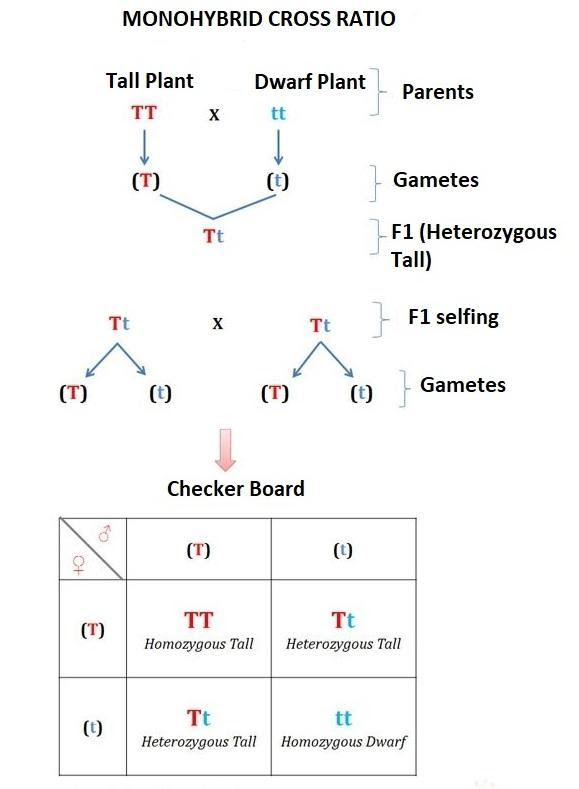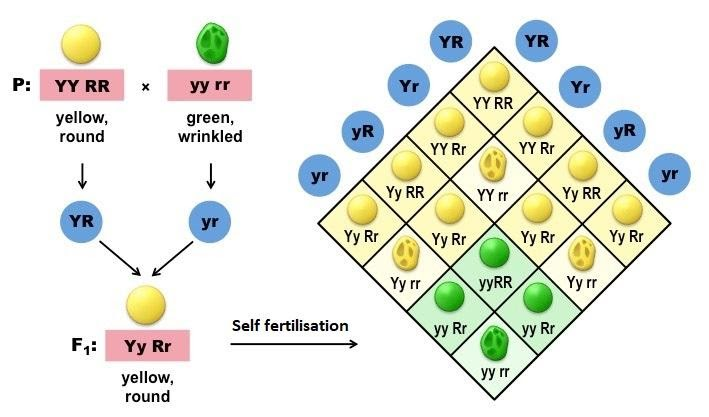Courses
Courses for Kids
Free study material
Offline Centres
MoreLast updated date: 09th Dec 2023
Total views: 280.8k
Views today: 8.80k

# A pure tall plant (TT) is crossed with a pure short plant (tt). The ratio of pure tall plant to pure short plant in ${F_2}$ generation will be?Verified
280.8k+ views
Hint: As we can see in the question, it is a monohybrid cross (when inheritance of only one character is observed). To find the ratio of ${F_2}$ generation, first we need to cross TT $\times$ tt and then self-cross of the hybrid of ${F_1}$ generation to get the progenies of ${F_2}$ generation. A pure tall plant means its allele should be genotypically homozygous, i.e., TT.

Mendel has chosen as pairs $14$ true pea varieties. One of them is the height of the plant recognized with the allele ‘T’ that represents the dominant condition ‘tall’ and the allele ‘t’ which is ‘dwarf’ and recessive.Monohybrid phenotypic ratio- $Tall:{\text{ }}Dwarf$= $\;3:{\text{ }}1$
Monohybrid genotypic ratio –$\;TT:{\text{ }}Tt:{\text{ }}tt$= $1:{\text{ }}2:{\text{ }}1$

Hence, A pure large plant with a pure, short plant is crossed (tt). In generation $F2$the ratio of pure large plants to pure short plants is $TT:{\text{ }}tt{\text{ }} = {\text{ }}1:1$.

Note:
The ratio of tall: dwarf plants would be$3:{\text{ }}1$. But here, in the question it has been asked to find the ratio of pure tall and pure dwarf plants. Out of these $3$tall plants, only one is pure homozygous (TT) tall and the other two are heterozygous (Tt) tall. So, the actual genotypic ratio is$TT:{\text{ }}Tt:{\text{ }}tt{\text{ }}-{\text{ }}1:2:1$.

Mendel laws:
Law of Dominance: It says that a dominant allele shows its effect in ${F_1}$generation in heterozygous condition and recessive allele fails to express itself in presence of dominant allele. It has exceptions of non-mendelian inheritances like codominance, incomplete dominance, etc.
Law of Segregation/ Law of Purity of gametes: According to this law, the alleles present in ${F_1}$generation segregate at the time of gamete formation, therefore gametes are pure for a particular trait. This law has no exception.
Law of Independent Assortment: This law cannot be seen in a monohybrid cross. The law states that when two pairs of hybrid features are combined, one pair is separated from another. Exception, connection.
DIHYBRID CROSSPhenotypic ratio: Round Yellow: Round green: wrinkled Yellow: wrinkled green
(R Y) : (R_yy) : (rrY) : (rryy)-
Nine : Three : Three : One

Genotypic ratio: RRYY: RRYy: RrYY: RrYy: RRyy: Rryy: rrYY: rrYy: rryy-
One : Two : Two : Four : One : Two : One : Two : One